### Home > INT3 > Chapter 8 > Lesson 8.1.1 > Problem8-8

8-8.
1. For each polynomial function below, make a table that includes integer x‑values from –2 to 2 and sketch each graph. Homework Help ✎

1. y = (x – 1)2(x + 1)

2. y = (x – 1)2(x + 1)2

3. y = x3 – 4x

4. Recall that the degree of a polynomial is the highest power of the variable when the polynomial is written in standard form. What is the degree of each polynomial in parts (a) through (c)?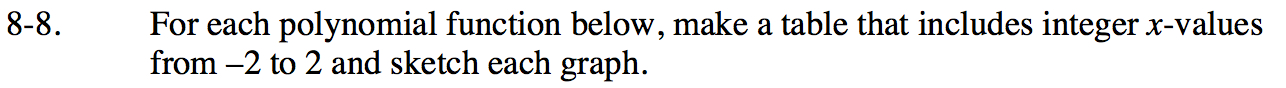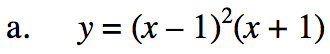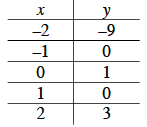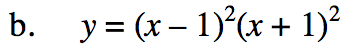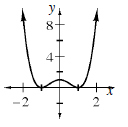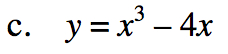Use the same method as in parts (a) and (b).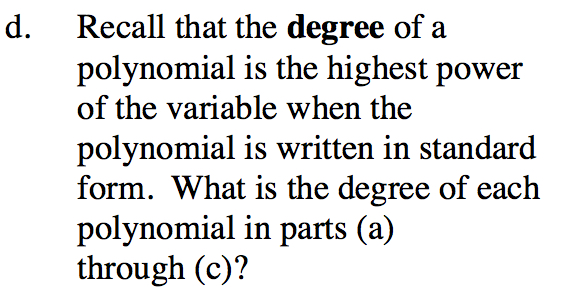Rewrite parts (a) and (b) in standard form.